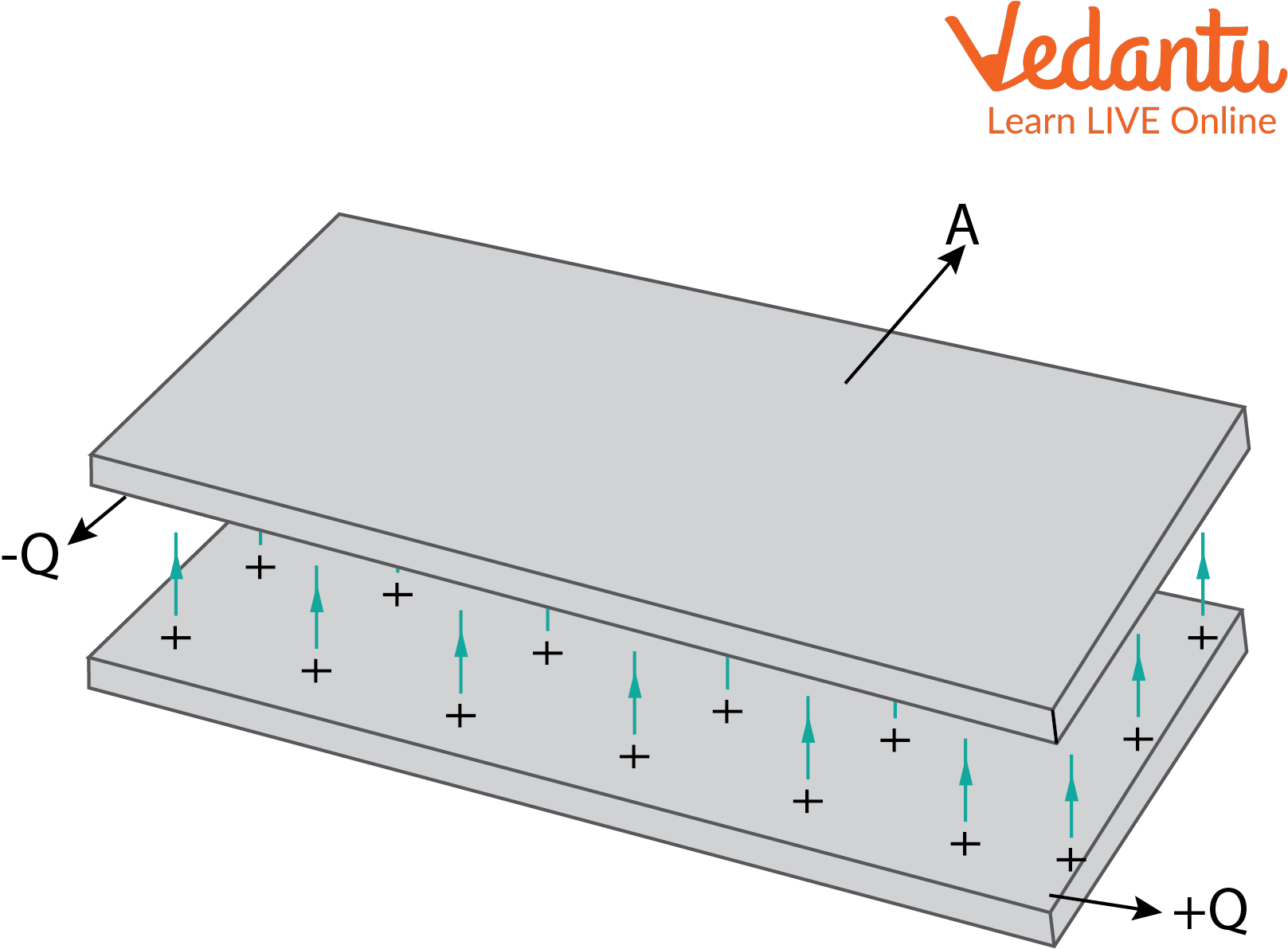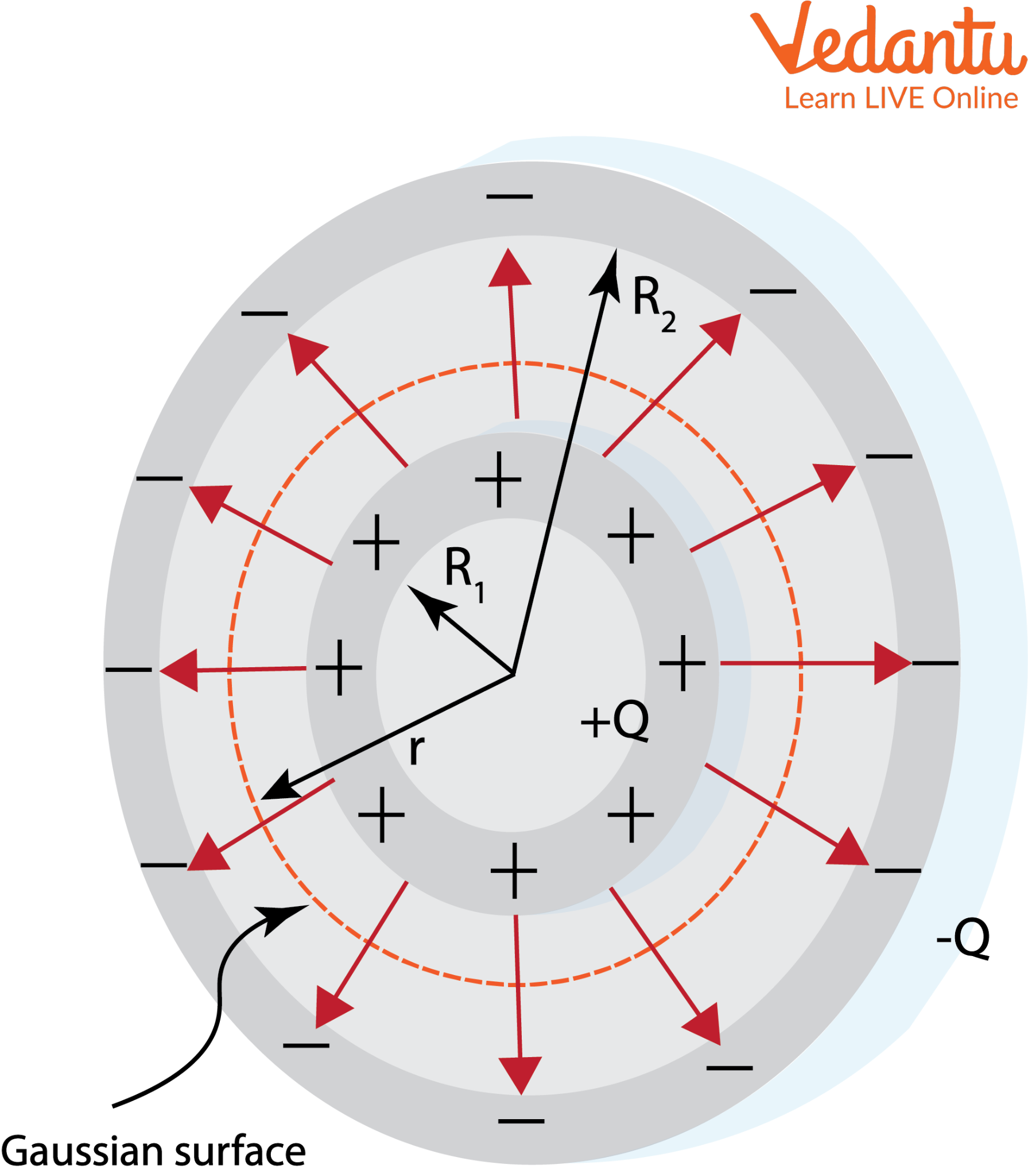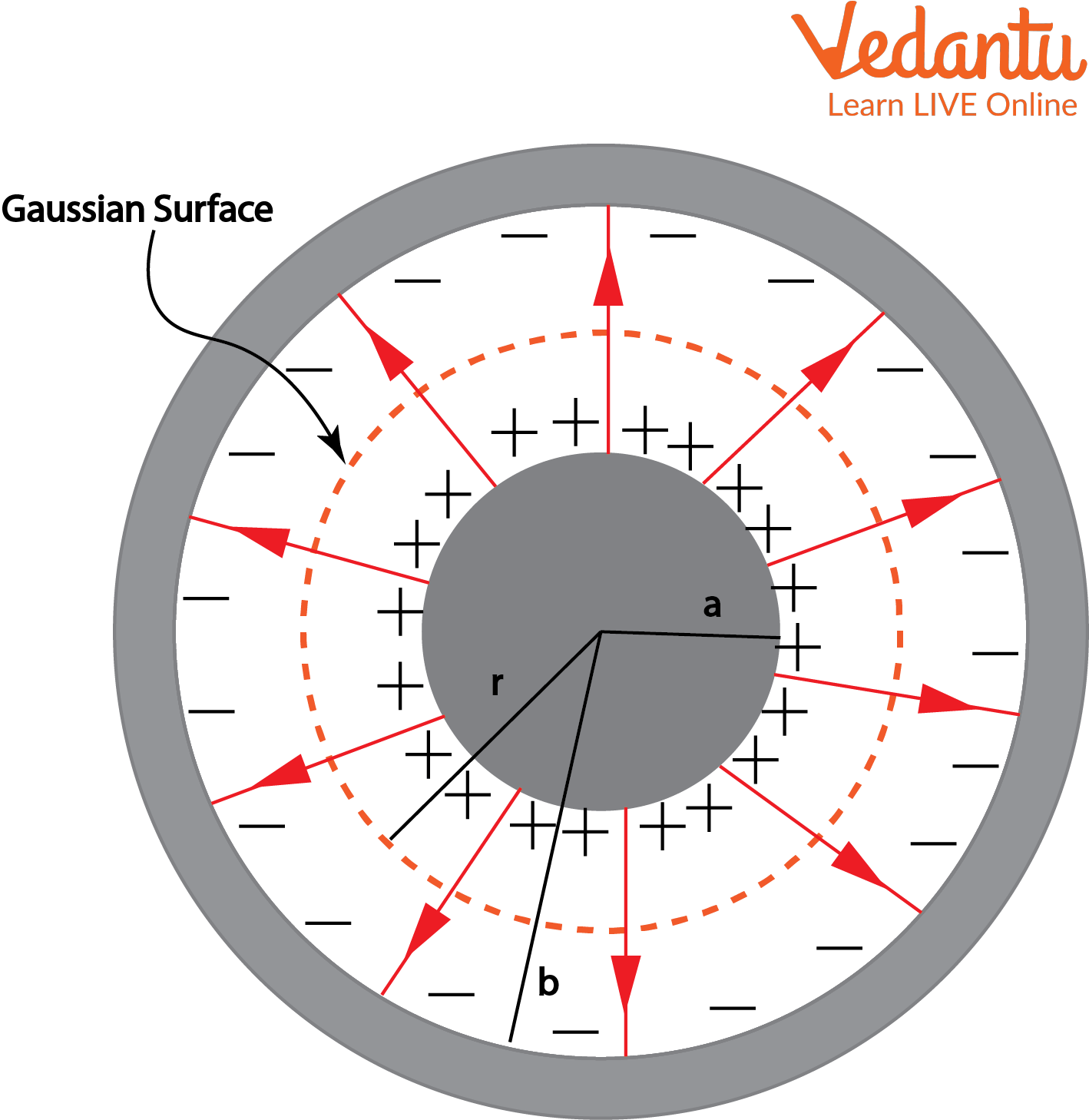Courses
Courses for Kids
Free study material
Offline Centres
More

# Spherical Capacitor and Parallel Plate Capacitor - JEE Important TopicLast updated date: 03rd Dec 2023
Total views: 21.6k
Views today: 1.21k## Capacitor and Capacitance

A capacitor is a kind of temporary battery in an electrical circuit. When it is connected to a charging source, the capacitor stores the electrical energy. Once the charging source is disconnected from the circuit, the capacitor starts to dissipate the stored energy.

An important measure in capacitors is capacitance. It can be defined as the ratio between the amount of electric charge stored in a conductor and the electric potential difference across the conductor. Capacitance can be acquired by self or mutually. It is denoted by the letter C and farad is the SI unit of capacitance. In this section, we will find the capacitance and energy stored in three main types of capacitors, namely parallel plate capacitor, spherical capacitor and cylindrical capacitor. The capacitance of spherical capacitor and the capacitance of cylindrical capacitor will be discussed in detail along with the expression of the energy stored in the capacitor.

## Expression for Energy Stored in Capacitor

Let’s say dV is the small amount of change in the potential difference. The corresponding change in its potential energy is

$dU = QdV$

Since Q =CV in a capacitor,

$dU = CVdV$

Integrating both sides of the equation,

$\int dU = \int CVdV$

$U = \dfrac{1}{2}CV^{2}$

$U = \dfrac{1}{2}(\dfrac{Q}{V})V^{2}$

$U = \dfrac{1}{2}QV$

$U = \dfrac{1}{2}Q\left ( \dfrac{Q}{C} \right )$

$U = \dfrac{1}{2}\dfrac{Q^{2}}{C}$

Hence, the equations for energy stored in a capacitor is

$U=\dfrac{1}{2}C{{V}^{2}}$

$=\dfrac{1}{2}QV$

$=\dfrac{{{Q}^{2}}}{2C}$

Now, let us discuss the energy stored in various capacitors.

## Capacitance and Energy Stored in a Parallel Plate Capacitor

A parallel plate capacitor consists of two parallel conducting plates of equal cross-section separated by a fixed distance d. There is no limitation on the shape of the capacitor. Let us consider a parallel plate capacitor carrying charges +Q and -Q, respectively, located at d from each other. The capacitor is connected to a battery.

The image below shows a parallel plate connector consisting of charges +Q and -Q. The plate taken in this case is a rectangular one.Parallel Plate Capacitor

When connected to a battery, due to the potential difference, one of the parallel plates gets positive charges while the other gets negative charges. Due to the potential difference between the charges in these two plates, an electric field gets induced between these plates as can be seen in the image above. The direction of this electric field is from the positively charged plate to the negatively charged plate.

The electric field in an individual plate can be expressed as:

$E = \dfrac{\sigma }{2\varepsilon _{o}}$

Where ${{\varepsilon }_{\circ }}$ is the permittivity in a vacuum (because it is considered that there is no medium between the capacitor plates) and σ is the charge density in the parallel plates. The net electric field is given by the sum of electric field intensity in both the plates.

Note that the magnitude of electric field intensity is the same in both the plates and the direction is also the same (positive to negative charge). Hence,

$E_{net} = \dfrac{\sigma }{\varepsilon _{o}}$

The charge density is nothing but the total charge divided by the cross-sectional area A of the plates. Therefore,

$E_{net} = \dfrac{Q}{A\varepsilon _{o}}$

The electric field intensity can also be expressed as a function of potential difference and the distance between the plates.

$E_{net} = \dfrac{V}{d}$

Now, comparing the two equations for net electric field intensity, we get

$\dfrac{V}{d}=\dfrac{Q}{A{{\varepsilon }_{o}}}$

$\dfrac{Q}{V}=\dfrac{A{{\varepsilon }_{o}}}{d}$

The ratio between charge stored and a unit potential difference is called capacitance. Hence,

$C = \dfrac{A\varepsilon _{o}}{d}$

So, now let us find the energy stored in parallel plate capacitor. We know that in parallel plate capacitor the charge $Q=\sigma ~A=K~E~A~ \varepsilon_o$ and capacitance $C = \dfrac{A\varepsilon _{o}}{d}$

Therefore, the energy stored in parallel plate capacitor will be:

$U =\dfrac{{{Q}^{2}}}{2C}=\dfrac {\left( K~E~A~ \varepsilon_o\right)^2}{2~\left(\dfrac{A\varepsilon _{o}}{d}\right)}$

$U=\dfrac {1}{2} ~K~{\varepsilon_o}~{E^2}~A$

## Capacitance of Spherical Capacitor and Energy Stored in a Spherical Capacitor

Spherical capacitors are formed by surrounding a solid/hollow spherical conductor with another concentric hollow spherical conductor. When connected to an energy source, both will be charged equally. But the potential difference exists because of the difference in the radius of the two spheres. The image below shows the cross-section of a spherical capacitor.Spherical Capacitor

Spherical capacitor derivation:

The electrical capacity of spherical conductor as per Gauss law is given by

$E = \dfrac{Q}{4\pi \epsilon _{o}r^{2}}$

The electric potential energy of a charged conductor is nothing but the line integral of the electric field. Hence the potential difference between two charged spheres separated by a distance is given by:

$V=-\int{E}dr$

$V=-\int_{{{R}_{2}}}^{{{R}_{1}}}{\dfrac{Q}{4\pi {{\varepsilon }_{o}}{{r}^{2}}}}$

$V=\dfrac{Q\left( {{R}_{2}}-{{R}_{1}} \right)}{4\pi {{\varepsilon }_{o}}{{R}_{1}}{{R}_{2}}}$

As we already know, capacitance is the charge divided by the potential difference. Hence,

$C = \dfrac{4\pi \varepsilon _{o}R_{1}R_{2}}{(R_{2}-R_{1})}$

It is evident from the above equation that the capacitance of a spherical conductor is dependent on the sphere radius and medium between the spheres.

After finding the capacitance of the spherical capacitor ,now let us move on to the energy stored in a spherical capacitor. The formula to find the energy stored in capacitors will be different for different capacitor types.

To find the energy stored in spherical capacitor, we need to the capacitance $C = 4~\pi ~{\varepsilon_o}~R$

Therefore, spherical capacitor formula:

$U =\dfrac{{{Q}^{2}}}{2C}=\dfrac {Q^2}{2~\left( 4~\pi ~{\varepsilon_o}~R\right)}$

$U=\dfrac {Q^2}{ 8~\pi ~{\varepsilon_o}~R}$

## Capacitance of Cylindrical Capacitor and Energy Stored in  a Cylindrical Capacitor

These capacitors consist of a hollow/solid cylindrical conductor enclosed by a hollow cylindrical conductor. They are used to store large amounts of electric charges in a small space. Let us consider a cylindrical capacitor of inner and outer radius a and b, respectively.Cylindrical Capacitor

Cylindrical capacitor derivation:

The Gaussian surface in this case is cylindrical with a length L. The electric field as per Gauss’s law is

$E = \dfrac{\lambda }{2\pi r\varepsilon _{o}}$

The potential difference across the two cylinders is given by

${{V}_{outer}}-{{V}_{inner}}=-\int_{a}^{b}{\dfrac{\lambda }{2\pi r{{\varepsilon }_{o}}}}dr$

${{V}_{outer}}-{{V}_{inner}}=-\dfrac{\lambda}{2\pi{{\varepsilon }_{o}}}\int_{a}^{b}{\dfrac{1}{r}}dr$

$=-\dfrac{\lambda }{2\pi {{\varepsilon }_{o}}}\left( ln\dfrac{b}{a} \right)$

As the outer cylinder is negatively charged, considering its voltage as zero, the potential difference can be obtained as

$V = \dfrac{\lambda }{2\pi \varepsilon _{o}}\left ( ln \dfrac{b}{a} \right )$

Now, the capacitance can be derived as follows:

$C=\dfrac{Q}{V}$

$C=\dfrac{\lambda L}{\dfrac{\lambda }{2\pi {{\varepsilon }_{o}}}\left( ln\dfrac{b}{a} \right)}$

$\dfrac{C}{L}=\dfrac{2\pi {{\varepsilon }_{o}}}{\left( ln\dfrac{b}{a} \right)}$

The above equation represents the capacitance per unit length of the cylindrical capacitor.

Then, the energy stored in the cylindrical capacitor:

$U =\dfrac{{{Q}^{2}}}{2C}$

Substitute the value of capacitance and simplify.

$U=\dfrac {Q^2}{\dfrac {2 L\pi {\varepsilon_o}}{\left( ln\dfrac{b}{a} \right)}}$

$U=\dfrac {Q^2}{2L\pi {\varepsilon_o}} ~\ln {\left(\dfrac {b}{a}\right)}$

The above equation is the formula to find the energy stored in a cylindrical capacitor.

## Applications of Capacitors

After learning about capacitors and their related information, now let us understand the applications or uses of capacitors. The following are a few of the major applications of capacitors:

• A large number of capacitors can be used in the audio systems of cars to provide excess energy required for amplifying sound.

• They are also used to condition the power supplies. In detail, due to their property of allowing AC and blocking DC signals during charging, they can easily split up these two signals and clean the power supply.

• They are used to reduce noise in a system.

• Capacitors can effectively counteract inductive loading caused by transmission lines in utility substations.

• Capacitors can also be used as sensors that can measure various parameters like humidity, mechanical strain, etc.

• Representation of binary information in the form of bits in Dynamic Random Access Memory (DRAM) is another exciting application of capacitors.

• They are used in combination with inductors for tuning circuits.

## Summary

Capacitors are devices used to store energy. When they are connected to the energy source, they charge themselves, and when the source is disconnected, they release the charges stored. Parallel plate capacitors, spherical capacitors, and cylindrical capacitors are the three most commonly used capacitor types. The capacitance is a measure of the amount of charge that can be stored and is the ratio of charge per unit potential difference. Energy stored in a capacitor is the work done in changing the potential difference by a small amount.

## FAQs on Spherical Capacitor and Parallel Plate Capacitor - JEE Important Topic

1. How will introducing a metal plate between the plates of a parallel plate capacitor affect its capacitance? Explain.

Introducing a metal plate in between the plates of a parallel plate capacitor will lead to infinite capacitance. The capacitance of the capacitor will depend directly on the permittivity of the medium in between. So, keeping all other variables constant, increasing the permittivity of the medium will increase the capacitance of a parallel plate capacitor. Also, the distance between the two plates will decrease leading to a redistribution of charges. As a result, both the plates will have the same potential. Hence, the capacitance increases.

2. How is capacitance related to energy stored in a capacitor?

The capacitance of capacitors are inversely proportional to the energy stored in them. It can be established using the following equation:

$U=\dfrac {Q^2}{2C}$